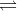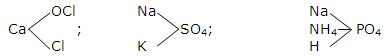Acid, Base, Neutralization, Salts for IIT JEE Chemistry

Properties of Salts for IIT JEE Main

Neutralization is the process in which acids and bases (alkali) are reacted which each and formed into salts and water. The process of neutralization involves the interaction between the OH- (which are basic/alkaline in nature) and H+ (which is acidic in nature) ions. When both the ions are reacted with each other then it results in the formation of water molecule.

For example, when strong acid such as hydrochloric acid (HCl) and strong base such as sodium hydroxide (NaOH) in aqueous solution of both the solution are interacted with each other in a specific amount, then Na+ ion from the NaOH and Cl- ion from the HCl are interacted with each other and together they form the salt named sodium chloride (NaCl); where H+ ion from the HCl and OH- ion from the NaOH are reacted with each other and converted to the water (H2O) molecule. The reaction between HCl and NaCl is shown below:

HCl + NaOH NaCl + H2O

The form salt sodium chloride does not possess any acidic as well basic property, both the property of the H+ ion and OH- ion are neutralized and eliminated during the reaction, hence the obtained salt NaCl sodium chloride is neutral in nature. So, such reaction is termed as acid base neutralisation reaction.

The hydrogen (H+) ion which mainly possesses the acidic properties further interacts with the basic property containing hydroxyl (OH-) ion and results in the neutral product water (H2O). The Na+ and CI- ions do not go under any chemical reaction and remains unchanged in the solution. On interacting with each other, they form into crystalline sodium chloride upon evaporation of the solution. Sodium chloride is categorized under the class of compounds named salts.

The result product of acid base neutralization in not always neutral in nature, although it involves the interaction between the hydrogen (H+) ion as well hydroxyl (OH-) ion which forms water (which is neutral in nature). The resulting solution nature is always dependent upon the concentration and type (strong/weak) of acid and base/alkali involved in the reaction. If the concentration of the acid is higher, then the resulting solution must be acidic in nature. Similarly if the concentration of alkali is higher, then the nature of the resulting solution must be basic in nature.

When equivalent amounts of acids and bases interact in the aqueous solution, the results can be demonstrated by several examples as follows:

• (i) When a strong acid (HCl) is reacted with a strong base (NaOH) it gives a neutral solution because both the acid as well base would be ionized completely and the reaction goes to completion.

• HCl + NaOHH2O + NaCl

• (ii) When a strong acid (HCl) is reacted with a weak base (NH4OH), it gives an acidic solution because the weak base such as NH4OH would not be ionized completely. Hence, the reaction does not go to completion and as the weak base is not ionized completely it results in the increase in the hydrogen ions in the solution.

• HCl+ NH4OHH2O + NH4Cl

• (iii) When a weak acid such as acetic acid (CH3COOH) is reacted with a strong base such as sodium hydroxide (NaOH) it gives a basic solution because the weak acid such as acetic acid (CH3COOH) is not ionized completely. Hence, the reaction does not go to completion and due to this the weak acid (acetic acid) is not ionized completely which results in the increase in the hydroxyl ions in the solution.

• CH3COOH + NaOHH2O + CH3COONa

• (iv) When a weak acid is reacted with a weak base it gives either an acidic or a basic or a neutral solution, which is dependent upon the relative strength of the weak acid as well as the weak base. And if the relative strength of both the weak acid and the weak base are equal then both the acid and base would be ionized completely and the reaction moves forward to its completion. Due to the equal strength of weak acid and weak base in the solution, the nature of the resulted solution must be neutral.

• CH3COOH + NH4OHH2O + CH3COONH4

Salts

All salts are mainly considered as ionic compounds which are mainly made up of the interaction between the positive and negative ions. In the salts, positive part of the ion comes from a base while negative part of the ion comes from an acid. Salts may contain different types of taste like salty, bitter, sweet, sour, astringent, tasteless etc. On the other hand, the solution prepared from salts may be acidic, basic or neutral in nature. Some salts such as fused salts and aqueous solution of each salt undergo electrolysis process and conduct electricity. Salts always exhibit the properties as ions in the aqueous form. In normal state, salts are crystalline solid materials.

Generally the salts are classified under four different classes as described below:

• (i) Simple salts:

• The simple salts are mainly obtained by the neutralization process. Neutralization is the process in which the acid and base are interacted with each other and termed into a simple salt. These simple salts are further sub-divided into three categories:

• a) Normal salts:

• The normal salts are also known as neural salts. These salts must be obtained because they loss their each possible proton (hydrogen atom or H+) from the structure. These salts are named as normal salts. Once the normal salts are formed, they cannot be separated easily. The normal salts are formed by the complete neutralization process.

These are the several examples of the normal salts: NaCl, NaNO3, K2SO4, Ca3(PO4)2, Na3BO3, Na2HPO3 (where the atom H is not replaced easily as the H3PO3 is a dibasic acid), NaH2PO2 (where both the H atoms are not replaced easily as H3PO2 is a monobasic acid), etc.

• b) Acid salts:

• The acid salts are formed by the process named incomplete neutralization between of the positive and negative ions. Here, the poly-basic acids are generally named as acid salts. Polybasic acid are the acids which contain two or more replaceable hydrogen atoms. These types of salts still possess one or more replaceable hydrogen atoms and when they come in contact with any base/ alkali, it results in the formation of acid salts. In other words, we can state that when polybasic acids are neutralised by alkali, they form acid salts.

The several examples of the acid salts are: NaHCO3, NaHSO4, NaH2PO4, Na2HPO4, etc.

• c) Basic salts:

• The basic salts are also formed by the process named incomplete neutralization between the positive and negative ions. Here, the poly-acidic bases are generally named as basic salts. Poly-acidic base is the base which contains two or more replaceable hydroxyl groups. These types of salts still possess one or more replaceable hydroxyl groups and when they come to contact with any acid, it results in the formation of basic salts. In other words, we can state that when poly-acidic bases are neutralised by acid, they form basic salts.

The several examples of basic salts are: Zn(OH)Cl, Mg(OH)Cl, Fe(OH)2Cl, Bi(OH)2Cl, etc.

• (ii) Double salts:

• Double salts are the salts which are made up of combination of two salts. Any two simple salts are combined with each other and formed into double salts. But such kinds of salts are stable only in the solid state.

The several examples of double salts are: Ferrous ammonium sulphate, FeS04-(NH4)2SO4.6H2O, Potash alum, K2SO4Al2(SO4)3.24H2O, and other alums.

Properties:

• a) When the double salt is dissolved in water, the ions of the salts that constitute it are furnished.

• b) The double salts solution must exhibit the properties of the simple salts from which they have been constituted.

• (iii) Complex salts:

• Complex salts are the salts which are made by the combination of simple salts or molecular compounds. Such kinds of salts are the most stable type of salts which are stable in the solid state as well as the liquid state. Here in the reaction, the obtained K4Fe(CN)and Co(NH3)6SO4 salts are the examples of the complex salts which are formed by the combination of simple salts or the molecular compounds.

FeSO4 + 6KCN→K4Fe(CN)+ K2SO4
CoSO4 + 6NH3 → Co(NH3)6SO4

Properties of Complex salts:

• a) When a complex salt is dissolves in water, it furnishes as a complex ion, the complex salt in water subdivides into two parts: positive and negative ions, from that one of the ion still remains in the complex form known as complex ion. In the following reaction the obtained [Fe(CN)6]4-and [Cu(NH3)4]2+ are the examples of the complex ions.

• K4F2(CN)6  ↔ 4K+ + [Fe(CN)6]4-
Cu(NH3)4SO4 ↔ [Cu(NH3)4]2+ +  SO4-2

• b) The solution property is different from the property of the substance form of which it has been constituted.

• (iv) Mixed salts:

• The mixed salt is a salts which furnishes more than one cation or anion when it is dissolved in the water.
The several examples of the mixed salts are: